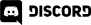# Home > Programming >

29. June 2017

Next, we need to create a bowling ball.  Instead of importing a 3D mesh, we are going to create one.  Select Game Object->3D Object->Sphere.Once created, rename it to BowlingBall.While we are here, create a new Tag called Ball and assign it to our object.

Let’s create a material for our Bowling ball.  Right click assets panel, select Create->Material.  Rename the newly created material BowlingBall.  Pick the color of your bowling ball with the box to the right of Albedo.  For shine add a small amount of Metallic as well.Now simply drag the newly created material over to our Bowling Ball in the scene.

We also need to attach physics to our bowling ball.  This time it’s using a Sphere Collider.  This is also a rigid body, but this time we increase the mass to 3.We are going to be putting all of the logic in our bowling ball.  One thing we are going to need to do is play audio when our ball hit’s a pin. We will add this logic later, but the audio source needs to be somewhere, so why not the bowling ball. We actually covered this process earlier when we added audio to our title screen.  Simply add an Audio Source component to the ball then attach the Hit.wav file, like so:Tick off the Play on Awake checkbox.

Now its time to add some scripting to our game to control input and to deal with physics collisions when they happen. Right click the Assets panel and create a new script like we did earlier. Rename it BowlingBall.cs and drag it onto our BowlingBall object.  Now double click our script and enter the following code:

```using System.Collections;
using System.Collections.Generic;
using UnityEngine;

public class BowlingBall : MonoBehaviour
{
public float force;
// Use this for initialization
private List<Vector3> pinPositions;
private List<Quaternion> pinRotations;
private Vector3 ballPosition;
void Start()
{
var pins = GameObject.FindGameObjectsWithTag("Pin");
pinPositions = new List<Vector3>();
pinRotations = new List<Quaternion>();
foreach (var pin in pins)
{
}

ballPosition = GameObject.FindGameObjectWithTag("Ball").transform.position;
}

// Update is called once per frame
void Update()
{
if (Input.GetKeyUp(KeyCode.Space))
if (Input.GetKeyUp(KeyCode.LeftArrow))
if (Input.GetKeyUp(KeyCode.RightArrow))
if (Input.GetKeyUp(KeyCode.R))
{
var pins = GameObject.FindGameObjectsWithTag("Pin");

for (int i = 0; i < pins.Length; i++)
{
//collision.gameObject.transform.parent.gameObject.tag
var pinPhysics = pins[i].GetComponent<Rigidbody>();
pinPhysics.velocity = Vector3.zero;
pinPhysics.position = pinPositions[i];
pinPhysics.rotation = pinRotations[i];
pinPhysics.velocity = Vector3.zero;
pinPhysics.angularVelocity = Vector3.zero;

var ball = GameObject.FindGameObjectWithTag("Ball");
ball.transform.position = ballPosition;
ball.GetComponent<Rigidbody>().velocity = Vector3.zero;
ball.GetComponent<Rigidbody>().angularVelocity = Vector3.zero;
}
}
if (Input.GetKeyUp(KeyCode.B))
{
var ball = GameObject.FindGameObjectWithTag("Ball");
ball.GetComponent<Rigidbody>().velocity = Vector3.zero;
ball.GetComponent<Rigidbody>().angularVelocity = Vector3.zero;
ball.transform.position = ballPosition;
}
if (Input.GetKeyUp(KeyCode.Escape))
{
Application.Quit();
}
}
private void OnCollisionEnter(Collision collision)
{
if (collision.gameObject.tag == "Pin")
GetComponent<AudioSource>().Play();
}}```

We publicly expose the Force value, which is the amount of energy to apply along the Z axis when we throw the ball.  Since it is public, it’s settable in the editor as a property in the script component.And done!